论文解读丨图神经网络应用于半结构化文档的命名实体识别和关系提取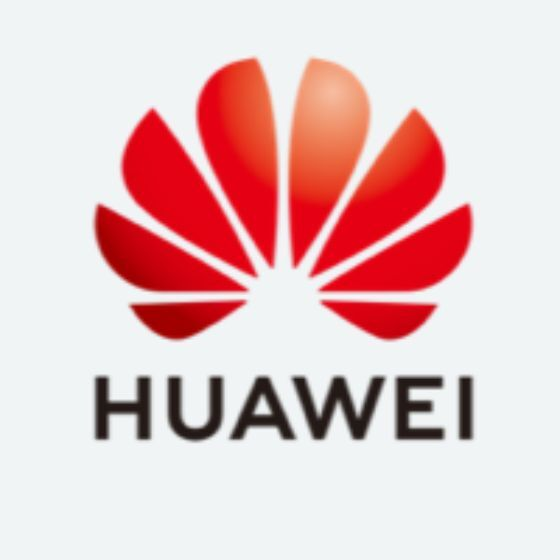​​​​​​摘要：随着用于传递和记录业务信息的管理文档的广泛使用，能够鲁棒且高效地从这些文档中自动提取和理解内容的方法成为一个迫切的需求。本次解读的文章提出利用图神经网络来解决半结构化文档中的实体识别（NER）和关系提取问题。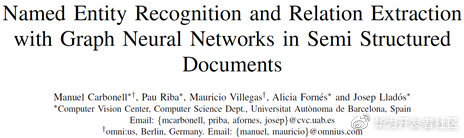1.方法

GNN 被广泛应用于 NER 和表格提取等任务中，本次解读的文章在此基础上提出将 GNN 应用于提取 key-value 对的任务中，不仅对文档图片中的实体进行分类，而且还会对实体间的关系进行预测。

（1）图的构造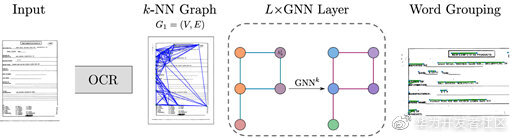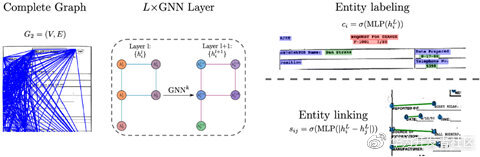（2）图的计算

\alpha_{ij}=\frac{exp⁡(LeakyRelu(V[Wh_i||Wh_j]))}{∑_{k \in N_{v_i}}exp⁡(LeakyRelu(V[Wh_i ||Wh_k]))}αij​=∑kNvi​​​exp⁡(LeakyRelu(V[Whi​∣∣Whk​]))exp⁡(LeakyRelu(V[Whi​∣∣Whj​]))​

h_i^{l+1}=g(h_i )=||_{k=1}^K\sigma(∑_{j \in N_i}α_{ij}^k W^k h_j^l )hil+1​=g(hi​)=∣∣k=1Kσ(jNi​∑​αijkWkhjl​)

c_i=σ(Wh_i^L)ci​=σ(WhiL​)

CE(y')=-(y∙log(y')+(1-y)∙log⁡(1-y' ))CE(y′)=−(ylog(y′)+(1−y)∙log⁡(1−y′))

2.实验结果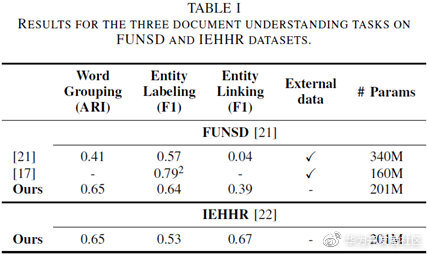评论